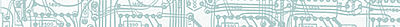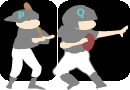# Disjunction

## Learn About Disjunction With The Following Examples And Interactive Exercises.Example 1:

 Given: p: Ann is on the softball team. q: Paul is on the football team. Problem: What does pq represent?Solution: In Example 1, statement p represents, "Ann is on the softball team" and statement q represents, "Paul is on the football team." The symbolis a logical connector which means "or." Thus, the compound statement pq represents the sentence, "Ann is on the softball team or Paul is on the football team." The statement pq is a disjunction.

Definition: disjunction is a compound statement formed by joining two statements with the connector OR. The disjunction "p or q" is symbolized by pq. A disjunction is false if and only if both statements are false; otherwise it is true. The truth values of pq are listed in the truth table below.

 p q pq T T T T F T F T T F F F

Example 2:

 Given: a: A square is a quadrilateral. b: Harrison Ford is an American actor. Problem: Construct a truth table for the disjunction "a or b."Solution:

 a b ab T T T T F T F T T F F F

Example 3:

 Given: r: x is divisible by 2. s: x is divisible by 3. Problem: What are the truth values of rs?

Solution: Each statement given in this example represents an open sentence, so the truth value of rs will depend on the replacement values of x as shown below.

If x = 6, then r is true, and s is true. The disjunction rs is true.

If x = 8, then r is true, and s is false. The disjunction rs is true.

If x = 15, then r is false, and s is true. The disjunction rs is true.

If x = 11, then r is false, and s is false. The disjunction rs is false.

Example 4:

 Given: p: 12 is prime. false q: 17 is prime. true r: 19 is composite. false Problem: Write a sentence for each disjunction below. Then indicate if it is true or false.
 1 pq 12 is prime or 17 is prime. true 2 pr 12 is prime or 19 is composite. false 3 qr 17 is prime or 19 is composite. true

Example 5: Complete a truth table for each disjunction below.

1.  a or b

2.  a or not b

3.  not a or b

 a b ab T T T T F T F T T F F Fa b ~b a~b T T F T T F T T F T F F F F T Ta b ~a ~ab T T F T T F F F F T T T F F T T

Students sometimes confuse conjunction and disjunction. Let's look at an example in which we compare the truth values of both of these compound statements.

Example 6:

 Given: x: Jayne played tennis. y: Chris played softball. Problem: Construct a truth table for conjunction "x and y" and disjunction "x or y."Solution:

 x y xy xy T T T T T F F T F T F T F F F F

With a conjunction, both statements must be true for the conjunction to be true; but with a disjunction, both statements must be false for the disjunction to be false. One way to remember this is with the following mnemonic: 'And’ points up to the sand on top of the beach, while ‘or’ points down to the ore deep in the ground.

Summary: A disjunction is a compound statement formed by joining two statements with the connector OR. The disjunction "p or q" is symbolized by pq. A disjunction is false if and only if both statements are false; otherwise it is true.

### Exercises

Directions: Read each question below. Select your answer by clicking on its button. Feedback to your answer is provided in the RESULTS BOX. If you make a mistake, choose a different button.

 1. Which of the following sentences is a disjunction? Amy played soccer or Bill played hockey.Amy played soccer and Bill played hockey.Amy did not play soccer and Bill played hockey.None of the above. RESULTS BOX:
 2. Which of the following statements is a disjunction? ~xyxyxyNone of the above. RESULTS BOX:
 3. A disjunction is used with which connector? AndOrNotNone of the above. RESULTS BOX:
 4. If a is false and b is true, what is the truth value of a~b? TrueFalseNot enough information was givenNone of the above. RESULTS BOX:
5.
 Given: r: y is prime. s: y is even. Problem: Which of the following is a true statement when y is replaced by 3?

r~s
r~s
rs
All of the above.

RESULTS BOX:

 Lessons on Symbolic Logic Negation Conjunction Disjunction Conditional Compound Biconditional Tautologies Equivalence Practice Exercises Challenge Exercises Solutions